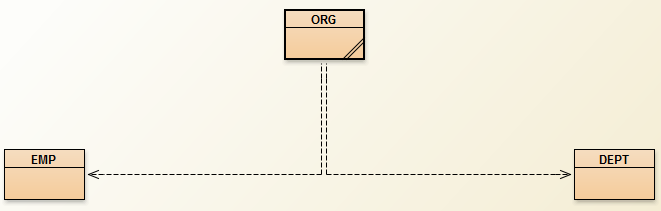Q:

# OOP java assignment1 jazan university

Q1) Study the following Class diagrams implemented in BlueJ IDE. The classes are:

1.      EMP

2.      DEPT

3.      ORG

A.     Write the implementation for the classes (EMP & DEPT). [Fields, Constructors, Methods].

B.     ORG class is the main class.

C.     Apply the following Object-Oriented Concepts through the implementation of the classes:

Ø  Accessor Methods.

Ø  Mutator Methods.

Ø  Internal Method call.

Ø  External Method call.

Ø  Variables Scope.

Ø  Creating Objects of one class inside other class.{ objects of EMP and DEPT must be created inside the main class ORG}.

Q2) Create a collection inside the class ORG, the collection must contains objects of class EMP. Apply the following actions:

Ø  Add 7 objects of EMP.

Ø  Remove the object at index 3

Ø  Print out all the objects

Ø  Check whether the collection is empty or not.

Ø   Replace objects 3 with object 1.``````public class EMP {

// Attributes
private int id;
private String name;
private double salary;
public static int counter = 0;

// Constructor
public EMP(String name, double salary) {
this.name = name;
this.salary = salary;
id = setId();
}
// Mutator methods
public String getName() {
return this.name;
}
public double getSalary() {
return salary;
}
public int getId(){
return id;
}
// Accessor methods
public void setName(String name) {
this.name = name;
}
public void setSalary(double salary) {
this.salary = salary;
}
public static int setId(){
counter++;
return counter;
}
public void print(){
System.out.println("Name : "+this.getName() +" Salary : "+ this.getSalary());
}
}``````

``````public class DEPT {

private int id;
private String name;

public int getId() {
return id;
}

public void setId(int id) {
this.id = id;
}

public String getName() {
return name;
}

public void setName(String name) {
this.name = name;
}
}
``````

``````import java.util.*;

public class ORG {

public static void main(String[] args) {

// TODO code application logic here
List<EMP> employees = new ArrayList<EMP>();
EMP e2 = new EMP("Ali",400000);
EMP e4 = new EMP("Maha",600000);
EMP e5 = new EMP("Salem",800000);
EMP e6 = new EMP("Jhon",1400000);
EMP e7 = new EMP("Rami",400000);

//Remove the object at index 3
employees.remove(3);
//print out all the objects
for(EMP e:employees){
e.print();
}
//check wether the collection is empty or not
if(employees.isEmpty())
System.out.println("the employees collection is empty");
else{
System.out.println("the employees collection is not empty");
}
//Replace objects 3 with object 1
employees.set(2,e1);
}
}``````